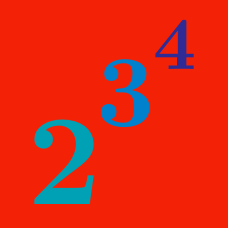Algebra

# Rules of Exponents: Level 2 Challenges

Which is larger

$\large A = 4^ { 3 ^ 2 } \quad \text{ or } \quad B = 2^ { 3 ^ 4 } ?$

Let $N$ be the number of 0's needed to write out $10^{100000}$.

How many 0's are needed to write out the number $N$?

$\Large \color{#3D99F6}{1!^{9!}} \hspace{7mm} \color{#20A900}{2!^{8!}} \hspace{7mm} \color{#EC7300}{3!^{7!}} \hspace{7mm} \color{#69047E}{4!^{6!}} \hspace{7mm} \color{#D61F06}{5!^{5!}}$

Which of the numbers above is the largest?

$\huge 27^{- \frac {x}{3} } + 81^{ \frac {1-x}{4} }$

If the expression above can be stated in the form of $\dfrac {a}{b^{^{\large{x}}}}$ for positive integers $a$ and $b$, what is the value of $a+b?$

Which is larger?

$\LARGE \color{#D61F06}{10}^{\color{#3D99F6}{7}} \quad \text{or} \quad \color{#3D99F6}{7}^{\color{#D61F06}{10}}$

×